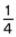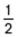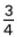# Year 4 programme of study

Number - number and place value

Pupils should be taught to:

• count in multiples of 6, 7, 9, 25 and 1,000
• find 1,000 more or less than a given number
• count backwards through 0 to include negative numbers
• recognise the place value of each digit in a four-digit number (1,000s, 100s, 10s, and 1s)
• order and compare numbers beyond 1,000
• identify, represent and estimate numbers using different representations
• round any number to the nearest 10, 100 or 1,000
• solve number and practical problems that involve all of the above and with increasingly large positive numbers
• read Roman numerals to 100 (I to C) and know that over time, the numeral system changed to include the concept of 0 and place value

Pupils should be taught to:

• add and subtract numbers with up to 4 digits using the formal written methods of columnar addition and subtraction where appropriate
• estimate and use inverse operations to check answers to a calculation
• solve addition and subtraction two-step problems in contexts, deciding which operations and methods to use and why

Number - multiplication and division

Pupils should be taught to:

• recall multiplication and division facts for multiplication tables up to 12 × 12
• use place value, known and derived facts to multiply and divide mentally, including: multiplying by 0 and 1; dividing by 1; multiplying together 3 numbers
• recognise and use factor pairs and commutativity in mental calculations
• multiply two-digit and three-digit numbers by a one-digit number using formal written layout
• solve problems involving multiplying and adding, including using the distributive law to multiply two-digit numbers by 1 digit, integer scaling problems and harder correspondence problems such as n objects are connected to m objects

Number - fractions (including decimals)

Pupils should be taught to:

• recognise and show, using diagrams, families of common equivalent fractions
• count up and down in hundredths; recognise that hundredths arise when dividing an object by 100 and dividing tenths by 10
• solve problems involving increasingly harder fractions to calculate quantities, and fractions to divide quantities, including non-unit fractions where the answer is a whole number
• add and subtract fractions with the same denominator
• recognise and write decimal equivalents of any number of tenths or hundreds
• recognise and write decimal equivalents to,,• find the effect of dividing a one- or two-digit number by 10 and 100, identifying the value of the digits in the answer as ones, tenths and hundredths
• round decimals with 1 decimal place to the nearest whole number
• compare numbers with the same number of decimal places up to 2 decimal places
• solve simple measure and money problems involving fractions and decimals to 2 decimal place

Measurement

Pupils should be taught to:

• convert between different units of measure [for example, kilometre to metre; hour to minute]
• measure and calculate the perimeter of a rectilinear figure (including squares) in centimetres and metres
• find the area of rectilinear shapes by counting squares
• estimate, compare and calculate different measures, including money in pounds and pence
• read, write and convert time between analogue and digital 12- and 24-hour clocks
• solve problems involving converting from hours to minutes, minutes to seconds, years to months, weeks to days

Geometry - properties of shapes

Pupils should be taught to:

• compare and classify geometric shapes, including quadrilaterals and triangles, based on their properties and sizes
• identify acute and obtuse angles and compare and order angles up to 2 right angles by size
• identify lines of symmetry in 2-D shapes presented in different orientations
• complete a simple symmetric figure with respect to a specific line of symmetry

Geometry - position and direction

Pupils should be taught to:

• describe positions on a 2-D grid as coordinates in the first quadrant
• describe movements between positions as translations of a given unit to the left/right and up/down
• plot specified points and draw sides to complete a given polygon

Statistics

Pupils should be taught to:

• interpret and present discrete and continuous data using appropriate graphical methods, including bar charts and time graphs
• solve comparison, sum and difference problems using information presented in bar charts, pictograms, tables and other graphs